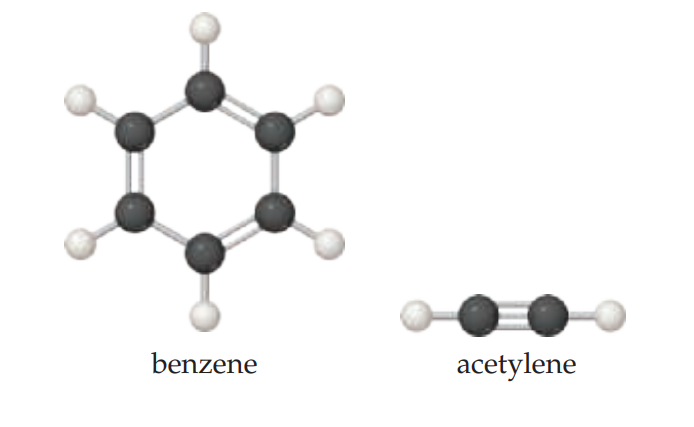×
Get Full Access to Chemistry: The Central Science - 14 Edition - Chapter 2 - Problem 2.46
Get Full Access to Chemistry: The Central Science - 14 Edition - Chapter 2 - Problem 2.46

×

# ?Ball-and-stick representations of benzene, a colorless liquid often used in organic chemistry reactions, and acetylene, a gas used as a fuel for high-ISBN: 9780134414232 1274

## Solution for problem 2.46 Chapter 2

Chemistry: The Central Science | 14th Edition

• Textbook Solutions
• 2901 Step-by-step solutions solved by professors and subject experts
• Get 24/7 help from StudySoup virtual teaching assistantsChemistry: The Central Science | 14th Edition

4 5 1 322 Reviews
20
0
Problem 2.46

Ball-and-stick representations of benzene, a colorless liquid often used in organic chemistry reactions, and acetylene, a gas used as a fuel for high-temperature welding, are shown below.

(a) Determine the molecular formula of each.

(b) Determine the empirical formula of each.Step-by-Step Solution:

Step 1 of 5) Theoretical and Percent Yields The quantity of product calculated to form when all of a limiting reactant is consumed is called the theoretical yield. The amount of product actually obtained, called the actual yield, is almost always less than (and can never be greater than) the theoretical yield. There are many reasons for this difference. Some of the reactants may not react, for example, or they may react in a way different from that desired (side reactions). In addition, it is not always possible to recover all of the product from the reaction mixture. The percent yield of a reaction relates actual and theoretical yields: One of the most important skills you can learn in school is how to think like a scientist. Questions such as: “What experiment might test this hypothesis”, “How do I interpret these data”, and “Do these data support the hypothesis” are asked every day by chemists and other scientists as they go about their work. We want you to become a good critical thinker as well as an active, logical, and curious learner. For this purpose, starting in this chapter, we include at the end of each chapter a special exercise called “Design an Experiment.” Here is an example: Is milk a pure liquid or a mixture of chemical components in water Design an experiment to distinguish between these two possibilities. You might already know the answer—milk is indeed a mixture of components in water—but the goal is to think of how to demonstrate this in practice. Upon thinking about it, you will likely realize that the key idea for this experiment is separation: Yo

Step 2 of 1

## Discover and learn what students are asking

Calculus: Early Transcendental Functions : Increasing and Decreasing Functions and the First DerivativeTest
?Using a Graph In Exercises 1 and 2, use the graph of to find (a) the largest open interval on which f is increasing, and (b) the largest open interval

Calculus: Early Transcendental Functions : Surface Integrals
?In Exercises 1 - 4, evaluate $$\int_{S} \int(x-2 y+z) d S$$. $$S: z=2, x^{2}+y^{2} \leq 1$$

Statistics: Informed Decisions Using Data : Organizing Qualitative Data
?What is a bar graph? What is a Pareto chart?

Unlock Textbook Solution